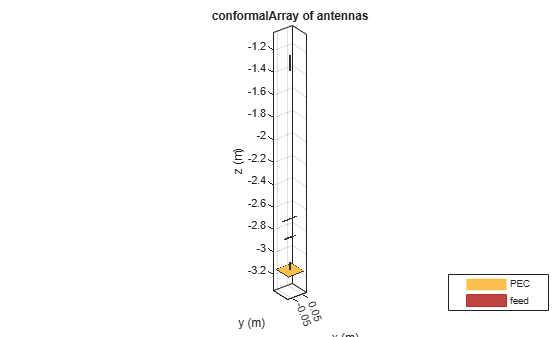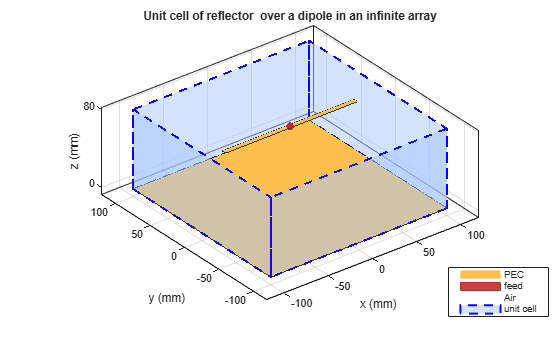# design

Design prototype antenna or arrays for resonance around specified frequency or create AI-based antenna from antenna catalog objects

## Syntax

``hant = design(antenna,frequency)``
``aiant = design(antenna,frequency,ForAI = true)``
``harray = design(array,frequency)``
``harray = design(array,frequency,elements)``
``harray = design(conformalarray,frequency)``
``harray = design(conformalarray,frequency,elements)``
``harray = design(infinitearray,frequency)``
``harray = design(infinitearray,frequency,elements)``
``ha = design(planewaveexcitation,frequency)``
``ha = design(planewaveexcitation,frequency,direction)``

## Description

example

````hant = design(antenna,frequency)` designs an `antenna` object from the antenna library that resonates at the specified `frequency`.```

example

````aiant = design(antenna,frequency,ForAI = true)` creates an Artificial Intelligence (AI) based antenna with a set of tunable properties which you can tune to explore the design space and perform AI-based analysis. NoteSupported antennas for this feature are: `dipole`Patch Antennas To use this feature, you need license to the Statistics and Machine Learning Toolbox™ in addition to the Antenna Toolbox™. ```
````harray = design(array,frequency)` designs an `array` of dipoles that operates at the specified frequency. The spacing between elements is half a wavelength.```

example

````harray = design(array,frequency,elements)` designs an array of elements for operation at the specified frequency. The spacing between elements is half a wavelength, if possible. If the object cannot achieve half-wavelength spacing, it uses the element size to calculate the separation between elements and evenly distributes the elements on a sphere with a radius proportional to the largest element in `element`.```
````harray = design(conformalarray,frequency)` designs a conformal array of dipole and bowtie elements at the specified frequency. The object places the elements in the locations specified by the default `conformalArray` object. If the object cannot place elements at the specified positions due to the intersection of elements, it uses the element size to calculate the spacing between elements and distributes the elements on a sphere with a radius proportional to the largest element in the `Elements`property of the `conformalArray` object.```
````harray = design(conformalarray,frequency,elements)` designs a conformal array of the specified elements at the specified frequency.```
````harray = design(infinitearray,frequency)` designs an infinite array with a reflector element at the specified frequency.```
````harray = design(infinitearray,frequency,elements)` designs an infinite array of the specified elements at the specified frequency. Note Antennas or arrays that you design with the `design` function resonate around the design frequency with an error tolerance of 10–15%. To reduce this tolerance and optimize your antenna or array design, use the `optimize` function.The `design` function uses air as its default substrate. ```
````ha = design(planewaveexcitation,frequency)` creates a plane-wave excitation environment and calculates the required orientation and polarization of the receiver antenna element to capture the maximum power from the incident plane-wave at the specified frequency.```
````ha = design(planewaveexcitation,frequency,direction)` creates a plane-wave excitation environment with the receiver antenna element orientation `direction` and calculates the required polarization of this antenna to capture the maximum power from the incident plane-wave at the specified frequency.```

## Examples

collapse all

Design a prototype microstrip patch antenna that resonates at a frequency of 1 GHz.

`p = design(patchMicrostrip,1e9)`
```p = patchMicrostrip with properties: Length: 0.1439 Width: 0.1874 Height: 0.0030 Substrate: [1x1 dielectric] GroundPlaneLength: 0.2998 GroundPlaneWidth: 0.2998 PatchCenterOffset: [0 0] FeedOffset: [0.0303 0] Conductor: [1x1 metal] Tilt: 0 TiltAxis: [1 0 0] Load: [1x1 lumpedElement] ```
`show(p)`Calculate the impedance of the above antenna at the same frequency.

`Z = impedance(p,1e9)`
```Z = 45.5212 - 9.1874i ```

Design a rectangular array of reflector backed rounded bowtie antennas to operate at 500 MHz.

```b = bowtieRounded(Tilt=90,TiltAxis=[0 1 0]); r = reflector(Exciter=b); ra = design(rectangularArray,500e6,r); show(ra)```Plot the radiation pattern of the rectangular array at 500 MHz.

`pattern(ra,500e6)`Create a default conformal array.

`confarraydef = conformalArray`
```confarraydef = conformalArray with properties: Element: {[1x1 dipole] [1x1 bowtieTriangular]} ElementPosition: [2x3 double] Reference: 'feed' AmplitudeTaper: 1 PhaseShift: 0 Tilt: 0 TiltAxis: [1 0 0] ```

Design a conformal array using a dipole antenna, folded dipole antenna, meander dipole antenna, and a monopole antenna at 1 GHz.

`desC = design(confarraydef,1e9,{dipole, dipoleFolded, dipoleMeander, monopole}) `
```desC = conformalArray with properties: Element: {[1x1 dipole] [1x1 dipoleFolded] [1x1 dipoleMeander] [1x1 monopole]} ElementPosition: [4x3 double] Reference: 'feed' AmplitudeTaper: 1 PhaseShift: 0 Tilt: 0 TiltAxis: [1 0 0] ```
`desC.ElementPosition`
```ans = 4×3 0 0 -1.3016 0 0 -2.6939 0 0 -2.8594 0 0 -3.1498 ```
`show(desC)`Create an infinite array.

`infarrayV1 = infiniteArray`
```infarrayV1 = infiniteArray with properties: Element: [1x1 reflector] ScanAzimuth: 0 ScanElevation: 90 RemoveGround: 0 ```
`show(infarrayV1)`Design the above array using a monopole antenna and at 1 GHz frequency.

`infarrayV2 = design(infarrayV1,1e9,monopole)`
```infarrayV2 = infiniteArray with properties: Element: [1x1 monopole] ScanAzimuth: 0 ScanElevation: 90 RemoveGround: 0 ```
`show(infarrayV2)`This example shows how to create an AI model based microstrip patch antenna at 1.67GHz and calculate its resonant frequency.

`pAI = design(patchMicrostrip,1.67e9,ForAI=true)`
```pAI = AIAntenna with properties: Antenna Info AntennaType: 'patchMicrostrip' InitialDesignFrequency: 1.6700e+09 Tunable Parameters Length: 0.0862 Width: 0.1122 Height: 0.0018 Use 'showReadOnlyProperties(pAI)' to show read-only properties ```

Vary its length and width and calculate its resonant frequency.

```pAI.Length = 0.0855; pAI.Width = 0.113; fR = resonantFrequency(pAI)```
```fR = 1.6806e+09 ```

Convert the `AIAntenna` to a regular microstrip patch antenna.

`pm = exportAntenna(pAI)`
```pm = patchMicrostrip with properties: Length: 0.0855 Width: 0.1130 Height: 0.0018 Substrate: [1x1 dielectric] GroundPlaneLength: 0.1795 GroundPlaneWidth: 0.1795 PatchCenterOffset: [0 0] FeedOffset: [0.0181 0] Conductor: [1x1 metal] Tilt: 0 TiltAxis: [1 0 0] Load: [1x1 lumpedElement] ```

## Input Arguments

collapse all

Antenna to design, specified as an antenna object from the Antenna Catalog.

Example: `dipole`

Array to design, specified as a `linearArray`, `rectangularArray`, or `circularArray` object.

Example: `rectangularArray`

Conformal array to design, specified as a `conformalArray` object.

You can position elements in a conformal array in three ways:

• Case 1: Points lie on a line.

• Case 2: Points lie on a plane.

• Case 3: Points lie in 3-D space.

Example: ```c = conformalArray;ca = design(c,50e6,{dipole,dipoleFolded, dipoleJ, bowtieTriangular,dipole,dipole,dipole,dipole,dipole});``` Designs a conformal array of specified elements operating at a frequency of 50 MHz.

Infinite array to design, specified as an `infiniteArray` object.

Example: `i = infiniteArray;ia = design(i,1e9,monopole);` Designs an infinite array with a monopole antenna element operating at a frequency of 1 GHz.

Plane-wave excitation environment, specified as a `planeWaveExcitation` object.

Example: `design(planeWaveExcitation,1e9);` Creates a plane-wave excitation environment and calculates the orientation and polarization of the receiver antenna required to capture the maximum power from the incident plane-wave at 1 GHz.

Resonant frequency of the antenna, specified as a real positive scalar.

Example: `55e6`

Data Types: `double`

Antenna object from the antenna library used in the array, specified as a single antenna element or a cell array in conformal array. For more information on element positions for conformal array, see `conformalarray`.

Example: ``` r = reflector;ra = design(rectangularArray,500e6,r); ```Designs a rectangular array of reflectors operating at a frequency of 500 MHz.

Example: ```c = conformalArray;ca = design(c,50e6,{dipole,dipoleFolded, dipoleJ, bowtieTriangular,dipole,dipole,dipole,dipole,dipole});``` Designs a conformal array of specified elements operating at a frequency of 50 MHz.

Orientation of the receiver antenna element, specified as a 1-by-3 vector of Cartesian coordinates or a 1-by-2 vector of azimuth and elevation angles. When you specify the Cartesian coordinates of a point, the function calculates the direction by joining a line from the origin to this point.

Example: ```design(planeWaveExcitation,1e9,[45 45])```

## Output Arguments

collapse all

Antenna object operating at the specified reference frequency, returned as an antenna object.

AI antenna object with set of tunable properties which you can tune to perform AI based analysis on this antenna.

Array object operating at the specified reference frequency and specified elements, returned as an array object.

Plane-wave excitation environment, returned as a `planeWaveExcitation` object. This output contains the receiver antenna orientation and polarization required to capture the maximum power from the incident plane-wave.

## Tips

• Use the `mesh` function to refine your antenna or array prototypes.

• Changing the design frequency in the `design` function changes the antenna or array element dimensions, ground plane dimensions, substrate thickness, feed location, spacing between the array elements, and array radius.

## Version History

Introduced in R2016b

expand all